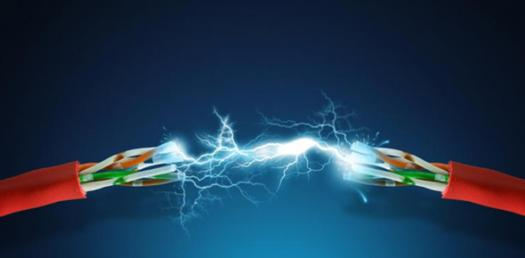# Electrical Set 2 Vol 1

70 Questions | Attempts: 130
ShareSettings.

• 1.
1. Light fixtures are classified by
• A.

The way they distribute light

• B.

The way they are installed

• C.

Their width and length

• D.

Their size and weight

• 2.
2. Which light classification directs from 40 to 60 percent of its light upward above the area to be lighted?
• A.

Indirect

• B.

Semi direct

• C.

Semi indirect

• D.

General diffusing

• 3.
3. Which classification of light fixture directs all its light so that the entire ceiling and upper side walls become the light source?
• A.

Indirect

• B.

Semi direct

• C.

Semi indirect

• D.

General diffusing

• 4.
4. What is the unit of measurement for lumens per square meter?
• A.

Lux

• B.

Foot candle

• C.

Candle power

• D.

Meter candle

• 5.
5. The efficiency range of a lamp is measure in lumens per
• A.

Volt

• B.

Ohm

• C.

Watt

• D.

Amp

• 6.
6. Incandescent lamps have efficiencies ranging from
• A.

1 to 6 lumens per watt

• B.

6 to 24 lumens per watt

• C.

24 to 60 lumens per watt

• D.

60 to 240 lumens watt

• 7.
7. What is the diameter of a T8 lamp?
• A.

8/2 inch

• B.

8/4 inch

• C.

8/8 inch

• D.

8/16 inch

• 8.
8. What frequency should you expect to find in electronic ballasts used in fluorescent lighting systems?
• A.

120 to 208 Hz

• B.

400 to 600 Hz

• C.

2,400 to 4,160 Hz

• D.

25,000 to 40,000 Hz

• 9.
What is used to power a light emitting diode (LED) array in a non-integrated LED fixture?
• A.

LED starter

• B.

LED driver

• C.

LED ballast

• D.

LED transformer

• 10.
10. What is a disadvantage of high intensity discharge (HID) lamps?
• A.

Low lumen per watt output

• B.

Lamp size is large

• C.

Lamp life is short

• D.

Restrike time

• 11.
11. What are the voltages that the 150 watt high pressure sodium (HPS) lamp comes in?
• A.

55 and 100 volts

• B.

55 and 220 volts

• C.

120 and 277 volts

• D.

100 and 48 volts

• 12.
12. What color light is associated with high pressure sodium (HPS) lamps?
• A.

Violet

• B.

Golden

• C.

Green blue

• D.

Blue white

• 13.
13. Which type of fixture is installed by laying it into the grid of a suspended ceiling?
• A.

Surface mount

• B.

Flush mount

• C.

Wall mount

• D.

Troffer

• 14.
14. When installing an octagon box for a new flush mount fixture what is important to ensure that the mounting method does not fail?
• A.

Box is sized to support the fixture

• B.

The fixture is less than 10 pounds

• C.

Fixture is secured using sheetrock screws

• D.

Fill capacity of the box to allow enough space for connections

• 15.
15. What are the two types of phase cut dimmers?
• A.

Forward and reverse

• B.

Analog and digital

• C.

Positive and negative

• D.

Single pole and double pole

• 16.
16. How does a phase cut dimmer operate?
• A.

A variable resistor is used to lower the voltage

• B.

Two or more resistors are used to form a voltage divider

• C.

The root mean square (RMS) voltage is reduced by turning off a portion of the input waveform

• D.

Semi conductor switches are used to turn off only the negative portion of the input waveform

• 17.
17. What is the light output of a fixture using a 0-10V ballast with 10 volts on the low voltage control circuit?
• A.

10%

• B.

50%

• C.

80%

• D.

100%

• 18.
18. What type of light fixture is typically used to illuminate aircraft on the parking apron?
• A.

500 watt floodlight

• B.

• C.

Low pressure sodium (LPS) light fixture

• D.

1000W -1500W high intensity discharge (HID) floodlight

• 19.
19. In an incandescent lamp, how much consumed energy is lost in the form of heat?
• A.

10%

• B.

50%

• C.

75%

• D.

90%

• 20.
20. What device is used in high intensity discharge (HID) lamps to limit current as the lamp heats up?
• A.

Ballast

• B.

Inductor

• C.

Resistor

• D.

Capacitor

• 21.
21. What letter represents the American National Standards Institute's special designation code for high pressure sodium (HPS) lamps?
• A.

S

• B.

H

• C.

L

• D.

M

• 22.
22. When installing fixtures, ensure uniform lighting by
• A.

Using only mercury vapor fixtures

• B.

Adjusting fixtures to 5 degree from horizontal

• C.

Using only high pressure sodium fixtures

• D.

Adjusting fixtures so that illuminated areas overlap

• 23.
23. To ensure that the proper amount of light is provided after fixture installation, check the lighting with
• A.

A stake

• B.

A voltmeter

• C.

A light meter

• D.

An ohmmeter

• 24.
24. When trouble shooting a 120 volt photocell, which conductor should provided power to the light fixture?
• A.

• B.

Line (black)

• C.

Neutral (white)

• D.

None of the above

• 25.
25. To prevent injury during re-lamping procedures, you must
• A.

Wear leather gloves

• B.

De-energize the light fixture

• C.

Wear approved safety goggles

• D.

Do all of the above tasks

## Related TopicsBack to top
×

Wait!
Here's an interesting quiz for you.